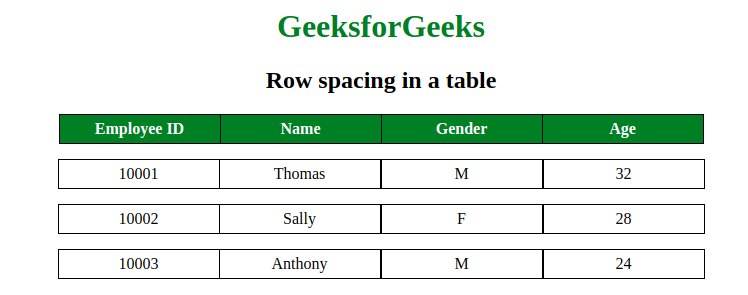# Space between two rows in a table using CSS?

• Last Updated : 10 May, 2022

The space between two rows in a table can be done using CSS border-spacing and border-collapse property. The border-spacing property is used to set the spaces between cells of a table and border-collapse property is used to specify whether the border of table is collapse or not. The border-spacing attribute can only be used if border-collapse attribute is set to separate. Example:

## html

 ```<``html``>``    ``<``head``>``        ``<``style``>``            ``table {``                ``border-collapse: collapse;``            ``}``            ``th {``                ``background-color:green;``                ``Color:white;``            ``}``            ``th, td {``                ``width:150px;``                ``text-align:center;``                ``border:1px solid black;``                ``padding:5px``            ` `            ``}``            ``.geeks {``                ``border-right:hidden;``            ``}``            ``.gfg {``                ``border-collapse:separate;``                ``border-spacing:0 15px;``            ``}``            ``h1 {``                ``color:green;``            ``}``        ````    ````    ``<``body``>``        ``<``center``>``        ``<``h1``>GeeksforGeeks``        ``<``h2``>Row spacing in a table``        ``<``table``>``            ``<``tr``>``                ``<``th``>Employee ID``                ``<``th``>Name``                ``<``th``>Gender``                ``<``th``>Age``            ````        ````        ``<``table` `class = "gfg">``            ``<``tr``>``                ``<``td` `class = "geeks">10001``                ``<``td``>Thomas``                ``<``td``>M``                ``<``td``>32``            ````            ``<``tr``>``                ``<``td` `class = "geeks">10002``                ``<``td``>Sally``                ``<``td``>F``                ``<``td``>28``            ````            ``<``tr``>``                ``<``td` `class = "geeks">10003``                ``<``td``>Anthony``                ``<``td``>M``                ``<``td``>24``            ````        ````        ````    `````

Output:CSS is the foundation of webpages, is used for webpage development by styling websites and web apps.You can learn CSS from the ground up by following this CSS Tutorial and CSS Examples.

My Personal Notes arrow_drop_up